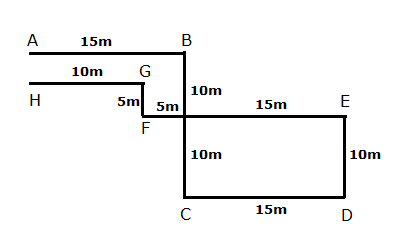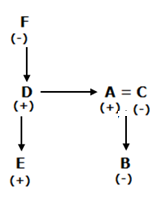# IBPS RRB Clerk/EPFO Assistant Prelims Reasoning Ability Questions 2019 (Day-7)

Dear Aspirants, Our IBPS Guide team is providing new series of Reasoning Questions for IBPS RRB Clerk/EPFO Assistant/LIC ADO Prelims Reasoning Ability Questions 2019 Prelims 2019 so the aspirants can practice it on a daily basis. These questions are framed by our skilled experts after understanding your needs thoroughly. Aspirants can practice these new series questions daily to familiarize with the exact exam pattern and make your preparation effective.

Check here for IBPS RRB Clerk Prelims Mock Test 2019

Check here for EPFO Assistant Prelims Mock Test 2019

[WpProQuiz 6664]

Directions (1 -3): Study the following information carefully and answer the given questions below

Anil walks 15m from point A towards the east to reach point B. Then he takes right turn and walks for 20m to reach point C. Now he takes a left turn and walks for 15m to reach point D. Then he takes a left turn and walks for 10m to reach point E. Then he takes a left turn and walks for 20m to reach point F. Now he takes a right turn and walks for 5m to reach point G. Then he takes a left turn and walks for 10m to reach point H.

1) If M is 5m to the north of G. then Point B is at what distance and in which direction with respect to point M?

a) 5m South

b) 10m West

c) 15m North

d) 5m, East

e) None of these

2) What is the distance between point H and Point A?

a) 10m

b) 5m

c) 9m

d) 15m

e) Cannot be determined

3) If Point Z is the midpoint between Point B and C, then which of the point will be nearest to Z?

a) A

b) E

c) D

d) F

e) None of these

Directions (4-6): Study the following information carefully and answer the questions given below.

B is the daughter of A. A is married to C. D is the brother of A. E is the only son of D. F is the grandmother of E. F has no daughter.

4) How C is related to E?

a) Uncle

b) Aunt

c) Nephew

d) Son-in law

e) None of these

5) If G is married to D, then how A is related to G?

a) Brother-in law

b) Brother

c) Sister in law

d) Mother in law

e) None of these

6) How B is related to F?

a) Grandson

b) Son

c) Granddaughter

d) Grandmother

e) None of these

Directions (7-10): In each of the following questions, relationship between different elements is shown in the statements followed by two conclusions. Find the conclusion which is definitely true.

7) Statements:

C ≥ A = T > E ≤ R; G > A ≥ M = H > K;

Conclusions:

I) H < C

II) C = H

a) Only II follows

b) Both I and II follows

c) Either I or II follows

d) Neither I nor II follows

e) Only I follow

8) Statements:

C ≥ A = T > E ≤ R; G > A ≥ M = H > K;

Conclusions:

I) G > R

II) C = H

a) Only II follows

b) Both I and II follows

c) Either I or II follows

d) Neither I nor II follows

e) Only I follow

9) Statements:

B ≥ M ≤ J ≥ K = L; S <M ≥ T > V ≥ W;

Conclusions:

I) S = J

II) J > S

a) Only II follows

b) Both I and II follows

c) Either I or II follows

d) Neither I nor II follows

e) Only I follow

10) Statements:

B ≥ M ≤ J ≥ K = L; S <M ≥ T > V ≥ W;

Conclusions:

I) W < B

II) V < K

a) Only II follows

b) Both I and II follows

c) Either I or II follows

d) Neither I nor II follows

e) Only I follow

Answers :

Directions (1 -3):1) Answer: D

2) Answer: B

3) Answer: D

Directions (4-6):

4) Answer: BC is the Aunt of E.

5) Answer: AA is the brother in law of G.

6) Answer: CB is the granddaughter of F.

Directions (7-10):

7) Answer: C

I) H < C —> C ≥ A ≥ M = H > K —> Can Be True

II) C = H—> C ≥ A ≥ M = H > K —> Can Be True

So either (i) or (ii) Follows

8) Answer: D

I) G > R —> G>A = T > E ≤ R —> False

II) C = H—> C ≥ A ≥ M = H > K —> Can Be True (But Not Definite)

So neither (i) or (ii) Follows

9) Answer: A

I) S =J—> S <M ≤ J ≥ K = L –> False

II) J > S—> S <M ≤ J ≥ K = L–> True

So, only (ii) follows

10) Answer: E

I) W < B —>B ≥ M ≥ T > V ≥ W –> True

II) V < K—>K ≤ J ≥ M ≥ T > V ≥ W –> False

So, Only (i) Follows

0 0 votes
Rating0 Comments
Inline Feedbacks
View all comments